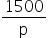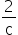Mathematics
Easy

Question

# The cost to rent a condominium at the beach is \$ 1500 per week. If two people share the cost, they each have to pay \$ 750. Write an inverse variation function that can be used to calculate the cost per person, c, of p persons sharing the rental fee## c =p =p = 750 × cp =Hint:

## The correct answer is: c =### Per person sharing is the price each guest pays during the contribution. Here we have given that the cost to rent a condominium at the beach is \$ 1500 per week. If two people share the cost, they each have to pay \$ 750. Lets look at the cost comparison per number of tenants, we have:For 1 person it is \$1500 per personFor 2 people it is \$750 per personFor 3 people it is \$500 per personHere we can clearly see that the cost per person reduces as the population grows. You are splitting the expense evenly if you are doing so. The rent is divided between the number of people= 1500/pSo c = 1500 / p

Here we have given that the cost to rent a condominium at the beach is \$ 1500 per week. If two people share the cost, they each have to pay \$ 750. So an an inverse variation function that can be used to calculate the cost per person, c, of p persons sharing the rental fee is c = 1500 / p

### Related Questions to study#### With Turito Foundation.#### Get an Expert Advice From Turito.